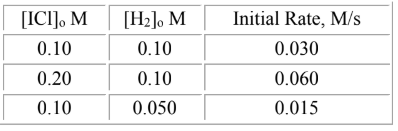# Problem: Iodine monochloride reacts with hydrogen according to the equation: 2 ICl(g) + H2(g) → I2(g) + 2 HCl(g). The rate data below have been obtained.Determine the rate law for the reaction.A. rate = k[ICl][H2] B. rate = k[ICl]2[H2]2C. rate = k[ICl]2[H2]D. rate = k[ICl][H2]1/2E. rate = k[ICl][H2]2

###### FREE Expert Solution

We're being asked to determine the rate law of the for the reaction using the data:

2 ICl(g) + H2(g) → I2(g) + 2 HCl(g)For this problem, follow the steps:

Step 1. Determine the order of ICl

Step 2. Determine the order of H2

Step 3. Setup the rate law equation

100% (56 ratings)###### Problem Details

Iodine monochloride reacts with hydrogen according to the equation: 2 ICl(g) + H2(g) → I2(g) + 2 HCl(g). The rate data below have been obtained.Determine the rate law for the reaction.

A. rate = k[ICl][H2

B. rate = k[ICl]2[H2]2

C. rate = k[ICl]2[H2]

D. rate = k[ICl][H2]1/2

E. rate = k[ICl][H2]2